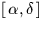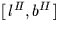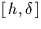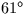Next: Formatting angles
Up: EXPLANATION AND EXAMPLES
Previous: EXPLANATION AND EXAMPLES

## Spherical Trigonometry

Celestial phenomena occur at such vast distances from the observer that for most practical purposes there is no need to work in 3D; only the direction of a source matters, not how far away it is. Things can therefore be viewed as if they were happening on the inside of sphere with the observer at the centre - the celestial sphere. Problems involving positions and orientations in the sky can then be solved by using the formulae of spherical trigonometry, which apply to spherical triangles, the sides of which are great circles.

Positions on the celestial sphere may be specified by using a spherical polar coordinate system, defined in terms of some fundamental plane and a line in that plane chosen to represent zero longitude. Mathematicians usually work with the co-latitude, with zero at the principal pole, whereas most astronomical coordinate systems use latitude, reckoned plus and minus from the equator. Astronomical coordinate systems may be either right-handed (e.g. right ascension and declination,Galactic longitude and latitude)or left-handed (e.g. hour angle and declination). In some cases different conventions have been used in the past, a fruitful source of mistakes. Azimuth and geographical longitude are examples; azimuth is now generally reckoned north through east (making a left-handed system); geographical longitude is now usually taken to increase eastwards (a right-handed system) but astronomers used to employ a west-positive convention. In reports and program comments it is wise to spell out what convention is being used, if there is any possibility of confusion.

When applying spherical trigonometry formulae, attention must be paid to rounding errors (for example it is a bad idea to find a small angle through its cosine) and to the possibility of problems close to poles. Also, if a formulation relies on inspection to establish the quadrant of the result, it is an indication that a vector-related method might be preferable.

As well as providing many routines which work in terms of specific spherical coordinates such as, SLALIB provides two routines which operate directly on generic spherical coordinates: sla_SEP computes the separation between two points (the distance along a great circle) and sla_BEAR computes the bearing (or position angle) of one point seen from the other. The routines sla_DSEP and sla_DBEAR are double precision equivalents. As a simple demonstration of SLALIB, we will use these facilities to estimate the distance from London to Sydney and the initial compass heading:

            IMPLICIT NONE

REAL D2R
PARAMETER (D2R=0.01745329252)

*  Longitudes and latitudes (radians) for London and Sydney
REAL AL,BL,AS,BS
PARAMETER (AL=-0.2*D2R,BL=51.5*D2R,AS=151.2*D2R,BS=-33.9*D2R)

*  Earth radius in km (spherical approximation)
REAL RKM
PARAMETER (RKM=6375.0)

REAL sla_SEP,sla_BEAR

*  Distance and initial heading (N=0, E=90)
WRITE (*,'(1X,I5,'' km,'',I4,'' deg'')')
:    NINT(sla_SEP(AL,BL,AS,BS)*RKM),NINT(sla_BEAR(AL,BL,AS,BS)/D2R)

END


(The result is 17011 km,.)

The routines sla_PAV and sla_DPAV are equivalents of sla_BEAR and sla_DBEAR but starting from direction-cosines instead of spherical coordinates.Next: Formatting angles
Up: EXPLANATION AND EXAMPLES
Previous: EXPLANATION AND EXAMPLES

SLALIB --- Positional Astronomy Library